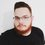# Exponential Diophantine Equation Troubles

Hello, fellow Brillianters, how are you all doing?

Recently I came across this question here, and whilst trying to come up with a proof for my solution, I stumbled upon this equation here:

$2*5^{a} - 1 = b^{2}$, where both a and b are non-negative integers.

I wanted to prove that there are only three pairs of solutions $(a,b)$ for this question; namely, $(0, 1)$, $(1, 3)$ and $(2, 7)$.

My first impulse was to try to prove that if any odd integer $b$, $b > 7$, is not a solution, then $b + 4$ cannot be a solution as well. I thought that it was sufficient until Calvin Lin came along and showed me that I only proved that $b$ and $b + 4$ cannot be solutions at the same time. Worst part is that he has no idea either of how to prove this.

So here I am, my friends; do you know a way to prove my statement right (or wrong)? I'd appreciate any form of help you can provide me. Thanks!Note by Alexandre Miquilino
5 years, 5 months ago

This discussion board is a place to discuss our Daily Challenges and the math and science related to those challenges. Explanations are more than just a solution — they should explain the steps and thinking strategies that you used to obtain the solution. Comments should further the discussion of math and science.

When posting on Brilliant:

• Use the emojis to react to an explanation, whether you're congratulating a job well done , or just really confused .
• Ask specific questions about the challenge or the steps in somebody's explanation. Well-posed questions can add a lot to the discussion, but posting "I don't understand!" doesn't help anyone.
• Try to contribute something new to the discussion, whether it is an extension, generalization or other idea related to the challenge.

MarkdownAppears as
*italics* or _italics_ italics
**bold** or __bold__ bold
- bulleted- list
• bulleted
• list
1. numbered2. list
1. numbered
2. list
Note: you must add a full line of space before and after lists for them to show up correctly
paragraph 1paragraph 2

paragraph 1

paragraph 2

[example link](https://brilliant.org)example link
> This is a quote
This is a quote
    # I indented these lines
# 4 spaces, and now they show
# up as a code block.

print "hello world"
# I indented these lines
# 4 spaces, and now they show
# up as a code block.

print "hello world"
MathAppears as
Remember to wrap math in $$ ... $$ or $ ... $ to ensure proper formatting.
2 \times 3 $2 \times 3$
2^{34} $2^{34}$
a_{i-1} $a_{i-1}$
\frac{2}{3} $\frac{2}{3}$
\sqrt{2} $\sqrt{2}$
\sum_{i=1}^3 $\sum_{i=1}^3$
\sin \theta $\sin \theta$
\boxed{123} $\boxed{123}$

Sort by:

This is a Ramanujan-Nagell type equation.

According to Wikipedia, a result of Siegel implies that the number of solutions in each case is finite, but not much further is known.

I believe It is unlikely that there is a simply proof of this statement.

Staff - 5 years, 5 months ago